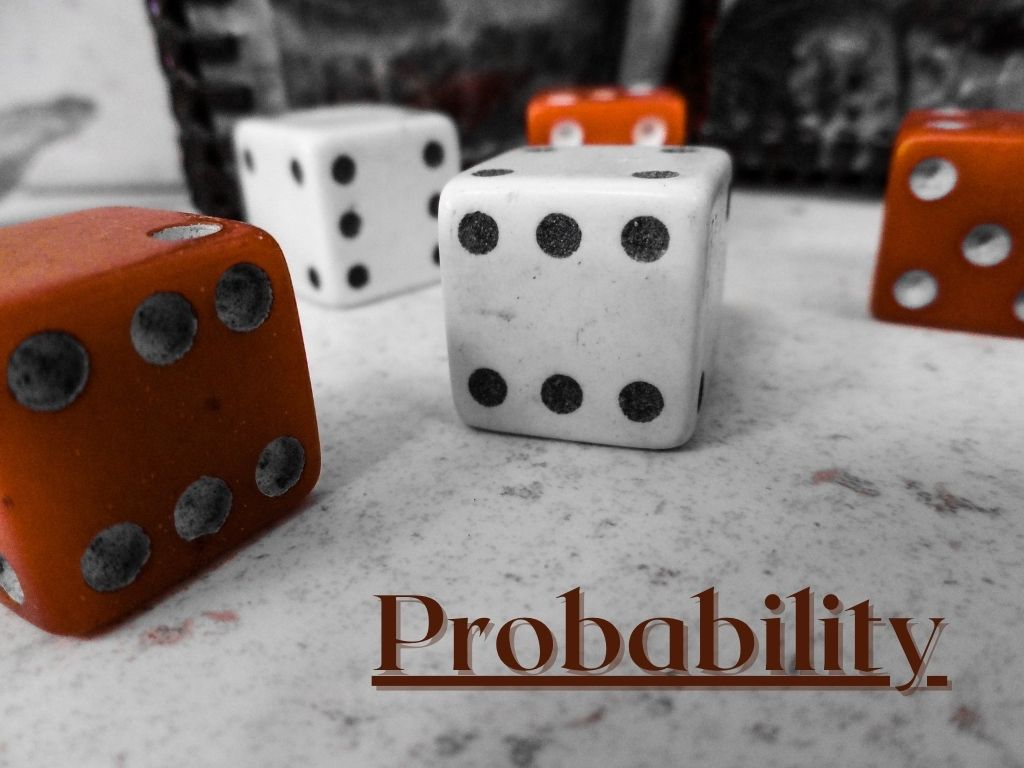## Probability Q & A

Q 1) What is probability? Ans. – Probability is the possibility of the occurrence of any event. When an event occurs, the chances of favorable outcomes are the value of probability. Q 2) What is the formula of probability? Ans. – Probability of an event = Number of favorable outcomes/total number of outcomes. For event …